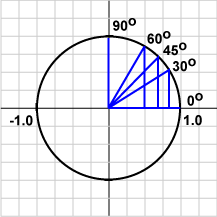```
a (u · v)  =  a |u| |v| cos θ

```

``` =   | au | |v| cos θ

=   (au) · v

=   u · (av)
```

# Cosine of 90°You may be somewhat fuzzy about how the cosine function behaves. Rather than memorize abstract stuff, visualize the unit circle with its radius projected onto the x-axis. From the picture,

the cosine of 0°   =   1.0,
the cosine of 30°   =   0.866,
the cosine of 45°   =   0.707,
the cosine of 60°   =   0.500,
the cosine of 90°   =   0.0.

Recall that:

u · v = |u| |v| cos θ

### QUESTION 5:

Two vectors are oriented at 90° to each other. What is their dot product?